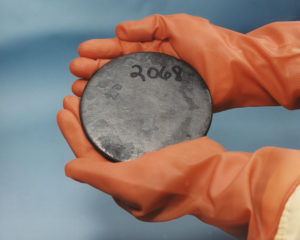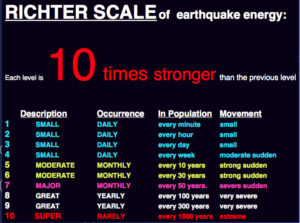## Applied Exponential and Logarithmic Equations

### Learning Outcomes

• Solve half-life problems
• Solve pH problems
• Solve problems involving Richter scale readings
• Solve problems involving decibels

In previous sections, we learned the properties and rules for both exponential and logarithmic functions. We have seen that any exponential function can be written as a logarithmic function and vice versa. We have used exponents to solve logarithmic equations and logarithms to solve exponential equations. We are now ready to combine our skills to solve equations that model real-world situations, whether the unknown is in an exponent or in the argument of a logarithm.One such application is called half-life, which refers to the amount of time it takes for half a given quantity of radioactive material to decay. The table below lists the half-life for several of the more common radioactive substances.

Substance Use Half-life
gallium-$67$ nuclear medicine $80$ hours
cobalt-$60$ manufacturing $5.3$ years
technetium-$99$m nuclear medicine $6$ hours
americium-$241$ construction $432$ years
carbon-$14$ archeological dating $5,715$ years
uranium-$235$ atomic power $703,800,000$ years

We can see how widely the half-lives for these substances vary. Knowing the half-life of a substance allows us to calculate the amount remaining after a specified time. We can use the formula for radioactive decay:

$\begin{array}{c}A\left(t\right)={A}_{0}{e}^{\frac{\mathrm{ln}\left(0.5\right)}{T}t}\hfill \\ A\left(t\right)={A}_{0}{e}^{\mathrm{ln}\left(0.5\right)\frac{t}{T}}\hfill \\ A\left(t\right)={A}_{0}{\left({e}^{\mathrm{ln}\left(0.5\right)}\right)}^{\frac{t}{T}}\hfill \\ A\left(t\right)={A}_{0}{\left(\frac{1}{2}\right)}^{\frac{t}{T}}\hfill \end{array}$

where

• ${A}_{0}$ is the amount initially present
• T is the half-life of the substance
• t is the time period over which the substance is studied
• $A(t)$ is the amount of the substance present after time t

### Example

How long will it take for ten percent of a $1000$-gram sample of uranium-$235$ to decay?

### Analysis of the Solution

Ten percent of $1000$ grams is $100$ grams. If $100$ grams decay, the amount of uranium-$235$ remaining is $900$ grams.

## pHIn chemistry, pH is used as a measure of the acidity or alkalinity of a substance. The pH scale runs from $0$ to $14$. Substances with a pH less than $7$ are considered acidic, and substances with a pH greater than $7$ are said to be alkaline. In our next example, we will find how doubling the concentration of hydrogen ions in a liquid affects pH.

### Example

In chemistry, $\text{pH}=-\mathrm{log}\left[{H}^{+}\right]$. If the concentration of hydrogen ions in a liquid is doubled, what is the effect on pH?

## Richter ScaleThe Richter Scale of earthquake energy.

The Richter scale is a logarithmic function that is used to measure the magnitude of earthquakes. The magnitude of an earthquake is related to how much energy is released by the quake. Instruments called seismographs detect movement in the earth; the smallest movement that can be detected shows on a seismograph as a wave with amplitude $A_{0}$.

A – the measure of the amplitude of the earthquake wave
$A_{0}$ – the amplitude of the smallest detectable wave (or standard wave)

From this you can find R, the Richter scale measure of the magnitude of the earthquake using the formula:

$R=\mathrm{log}\left(\frac{A}{A_{0}}\right)$

The intensity of an earthquake will typically measure between $2$ and $10$ on the Richter scale. Any earthquakes registering below a $5$ are fairly minor; they may shake the ground a bit but are seldom strong enough to cause much damage. Earthquakes with a Richter rating between $5$ and $7.9$ are much more severe, and any quake above an $8$ is likely to cause massive damage. (The highest rating ever recorded for an earthquake is $9.5$ during the $1960$ Valdivia earthquake in Chile.)

### Example

An earthquake is measured with a wave amplitude $392$ times as great as $A_{0}$. What is the magnitude of this earthquake, to the nearest tenth, using the Richter scale?

A difference of $1$ point on the Richter scale equates to a $10$-fold difference in the amplitude of the earthquake (which is related to the wave strength). This means that an earthquake that measures $3.6$ on the Richter scale has $10$ times the amplitude of one that measures $2.6$.

In the Richter scale example, the wave amplitude of the earthquake was $392$ times the smallest detectable wave. What if it were $10$ times that or $3,920$ times normal? To find the measurement of that size earthquake on the Richter scale, you find log $3920$. A calculator gives a value of $3.5932…$ or $3.6$ when rounded to the nearest tenth. One extra point on the Richter scale can mean a lot more shaking!

In the following video, we show another example of how to calculate the magnitude of an earthquake.

## DecibelsLogarithms can be used to measure how loud sound is.

Sound is measured in a logarithmic scale using a unit called a decibel. The formula looks similar to the Richter scale:

$d=10\mathrm{log}\left(\frac{P}{P_{0}}\right)$

where P is the power or intensity of the sound and$P_0$ is the weakest sound that the human ear can hear. In the next example we will find how much more intense the noise from a dishwasher is than the noise from a hot water pump.

### Example

One hot water pump has a noise rating of $50$ decibels. One dishwasher, however, has a noise rating of $62$ decibels. The dishwasher noise is how many times more intense than the hot water pump noise?

The following video provides another example of comparing the intensity of two sounds.

Applications of logarithms and exponentials are everywhere in science. We hope the examples here have given you an idea of how useful they can be.